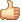# java提高篇（十三）—–equals()方法总结

## equals()

``````public boolean equals(Object obj) {
return (this == obj);
}
``````

``````public boolean equals(Object anObject) {
if (this == anObject) {
return true;
}
if (anObject instanceof String) {
String anotherString = (String)anObject;
int n = count;
if (n == anotherString.count) {
char v1[] = value;
char v2[] = anotherString.value;
int i = offset;
int j = anotherString.offset;
while (n-- != 0) {
if (v1[i++] != v2[j++])
return false;
}
return true;
}
}
return false;
}
``````

equals 方法在非空对象引用上实现相等关系：

• 1、自反性：对于任何非空引用值 x，x.equals(x) 都应返回 true。
• 2、对称性：对于任何非空引用值 x 和 y，当且仅当 y.equals(x) 返回 true 时，x.equals(y) 才应返回 true。
• 3、传递性：对于任何非空引用值 x、y 和 z，如果 x.equals(y) 返回 true，并且 y.equals(z) 返回 true，那么 x.equals(z) 应返回 true。
• 4、一致性：对于任何非空引用值 x 和 y，多次调用 x.equals(y) 始终返回 true 或始终返回 false，前提是对象上 equals 比较中所用的信息没有被修改。
• 5、对于任何非空引用值 x，x.equals(null) 都应返回 false。

• 1) 对象域，使用equals方法 。
• 2) 类型安全的枚举，使用equals或== 。
• 3) 可能为null的对象域 : 使用 == 和 equals 。
• 4) 数组域 : 使用 Arrays.equals 。
• 5) 除float和double外的原始数据类型 : 使用 == 。
• 6) float类型: 使用Float.foatToIntBits转换成int类型，然后使用==。
• 7) double类型: 使用Double.doubleToLongBit转换成long类型，然后使用==。

``````public boolean equals(Object obj) {
return (obj instanceof Float)
&& (floatToIntBits(((Float)obj).value) == floatToIntBits(value));
}
``````

``````However, there are two exceptions:
If f1 and f2 both represent
Float.NaN, then the equals method returns
true, even though Float.NaN==Float.NaN
has the value false.
If <code>f1 represents +0.0f while
f2 represents -0.0f, or vice
versa, the equal test has the value
false, even though 0.0f==-0.0f
has the value true.
``````

## 在equals()中使用getClass进行类型判断

``````public class Person {
protected String name;

public String getName() {
return name;
}

public void setName(String name) {
this.name = name;
}

public Person(String name){
this.name = name;
}

public boolean equals(Object object){
if(object instanceof Person){
Person p = (Person) object;
if(p.getName() == null || name == null){
return false;
}
else{
return name.equalsIgnoreCase(p.getName());
}
}
return false;
}
}
``````

``````public class Employee extends Person{
private int id;

public int getId() {
return id;
}

public void setId(int id) {
this.id = id;
}

public Employee(String name,int id){
super(name);
this.id = id;
}

/**
* 重写equals()方法
*/
public boolean equals(Object object){
if(object instanceof Employee){
Employee e = (Employee) object;
return super.equals(object) && e.getId() == id;
}
return false;
}
}
``````

``````public class Test {
public static void main(String[] args) {
Employee e1 = new Employee("chenssy", 23);
Employee e2 = new Employee("chenssy", 24);
Person p1 = new Person("chenssy");

System.out.println(p1.equals(e1));
System.out.println(p1.equals(e2));
System.out.println(e1.equals(e2));
}
}
``````

### 评论 13

1. #9

收获很多，谢谢。站在巨人的肩膀上学的很快！

别忘微笑2年前 (2017-07-31)回复
2. #8

thank you

java欧尼酱2年前 (2017-07-21)回复
3. #7

thank you

无双未央2年前 (2017-07-21)回复
4. #6

aaaa

java欧尼酱2年前 (2017-07-21)回复
5. #5

aaaa

无双未央2年前 (2017-07-21)回复
6. #4

6) float类型: 使用Float.foatToIntBits转换成int类型，然后使用==。 7) double类型: 使用Double.doubleToLongBit转换成long类型，然后使用==。 还是不明白为啥这两不能用==

Kyle3年前 (2016-08-22)回复
• 这个东西会比较复杂。涉及到底层知识：原码、反码、补码、移码的问题。http://blog.csdn.net/turkeyzhou/article/details/2755970，你可以看看这篇博客

selfImpro3年前 (2016-08-22)回复
•大神V5

Kyle3年前 (2016-08-23)回复
7. #3

我一般是这么重写equals方法的：public boolean equals(Object o) { if(o == null) { return false; } if (o == this) { return true; } if (this.getClass() != o.getClass()) { return false; } Employee e = (Employee) o; return (this.getId() == e.getId() && this.firstname.equals(e.firstname) && this.lastName.equals(e.lastName));}

yusijia3年前 (2016-06-29)回复
• equals方法重写有很多技巧和需要主要的地方。一定要注意equals的五个规则

selfImpro3年前 (2016-06-30)回复
8. #2

子类覆写父类equals时用getClass，否则极易出现Person例子中p1.equals(e1)为true，反之为false的情况，而当由超类决定相等关系时，用instanceof即可

TT非5年前 (2014-08-04)回复
9. #1

这个网站非常好。。我看的java提高篇。收货很多

董爷5年前 (2014-05-10)回复
• 非常荣幸能够给与你帮助

chenssy5年前 (2014-05-10)回复

## Java 技术驿站 | 致力打造 Java 精品博客

### 觉得文章有用就打赏一下文章作者

#### 支付宝扫一扫打赏#### 微信扫一扫打赏